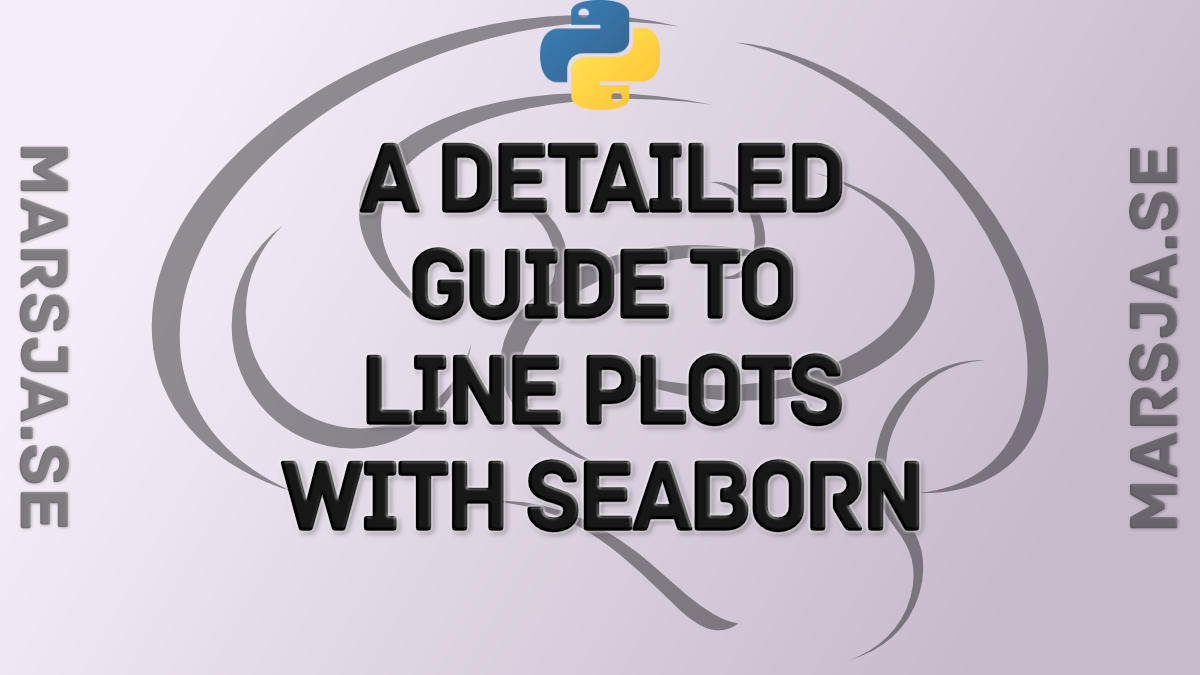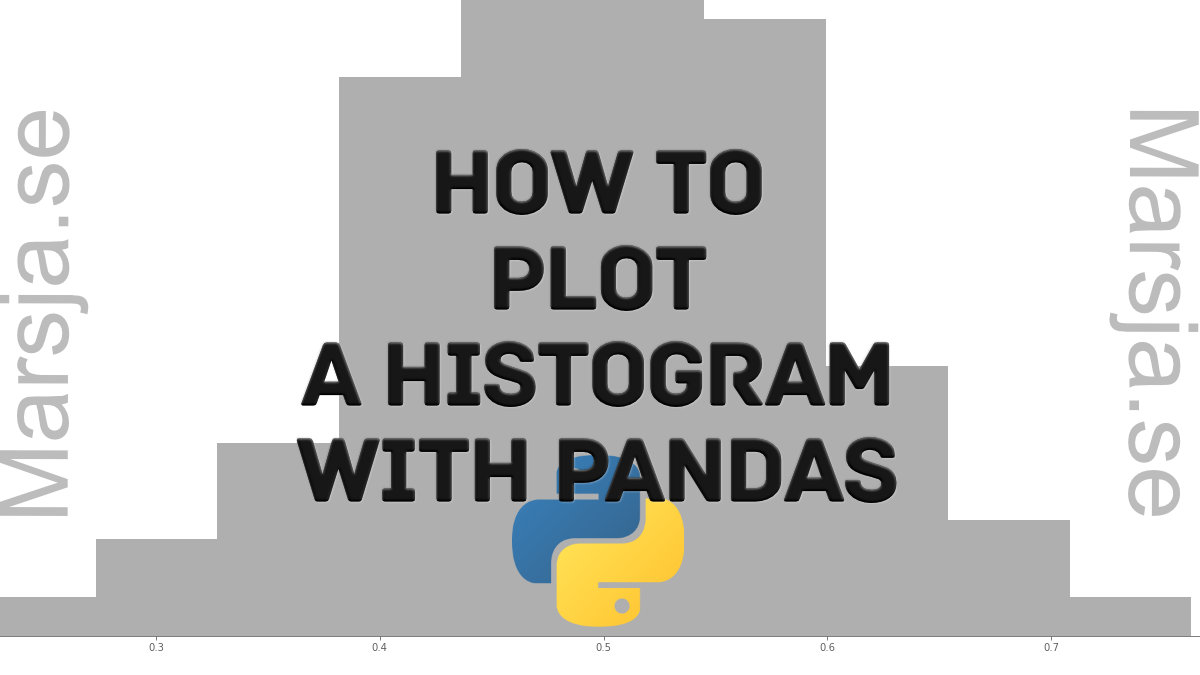## Seaborn Line Plots: A Detailed Guide with Examples (Multiple Lines)

In this Python data visualization tutorial, we will learn how to create line plots with Seaborn. First, we’ll start with the simplest example (with one line) and then we’ll look at how to change the look of the graphs, and how to plot multiple lines, among...## How to Plot a Histogram with Pandas in 3 Simple Steps

In this post, we are going to learn how to plot histograms with Pandas in Python. Specifically, we are going to learn 3 simple steps to make a histogram with Pandas. Now, plotting a histogram is a good way to explore the distribution of our data. Note, at the end of...